# Analysis Of LeoFinace and Solana TokenI have been releasing subsequent analysis between Leo token and other tokens and alt coins such as bitcoin, ethereum and even binance.

So far so good it indicated a minimum positive correlation for bitcoin and ethereum even for binance so that of binance wasn’t a result to be dependent on.

In the course of this article I shall analyze the relationship between that of Leo token and Solana.Image Source - coinmarketcap com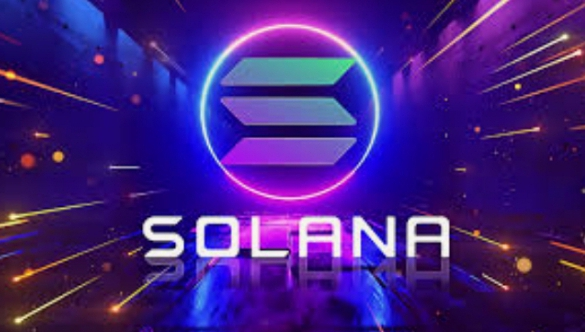Image Source - medium.com

Still in the course of this article, I shall cover up for the following basics of correlation analysis to have an understanding on how the result is been interpreted.

Here are the points I intend to cover, what correlation analysis is about, theories of correlation, correlation coefficient, null hypothesis correlation analysis , collection of data from coin gecko, analysis of scatter plot diagram and interpretation of result.

What correlation analysis is about.

Long time ago an statistician introduced the term correlation to find out relationship between variables which shall be considered.

The relationship between that of leo token and that of Solana will be measured from the duration of April 23, 2022 – May 23, 2022.

Positive correlation
A positive correlation indicates that increase in one variable, the higher the other variable in value, the simultaneous increase in other variable. This entails the coefficient of correlation is positive as well and tends towards a positive one axis on the Pearson number scale system.

Negative correlation
A negative correlation indicates that when an increase in one will lead to a simultaneous decrease in the other as both variable as they occur on a single process. Such correlation is said to be a negative one and tends towards -1 on the Pearson number scale system.

Zero correlation
Zero correlation indicates that there is no relationship at all between the variables in consideration and the correlation coefficient on a number line tends towards zero from the direction of either positive or negative ones.

Pearson represented the three intervals of correlation on a number scale.

Hypothesis for correlation analysis

Hypothesis is a statement of fact that generalizes a data based on concerned question.

Null Hypothesis for correlation
The null hypothesis correlation analysis states that there is no relationship between variables of consideration or concern. Whereas the opposite of null hypothesis is the alternate hypothesis test.

The coefficient of correlation resides on a line of -1 to 0 to +1 as seen below.
-1______________0______________+1

Collection of data from coin gecko between the price of Leo token and that of Solana token was carried out using spss data entry software.

The data was collected using coin gecko within the period of April, 23 – May, 23 2022.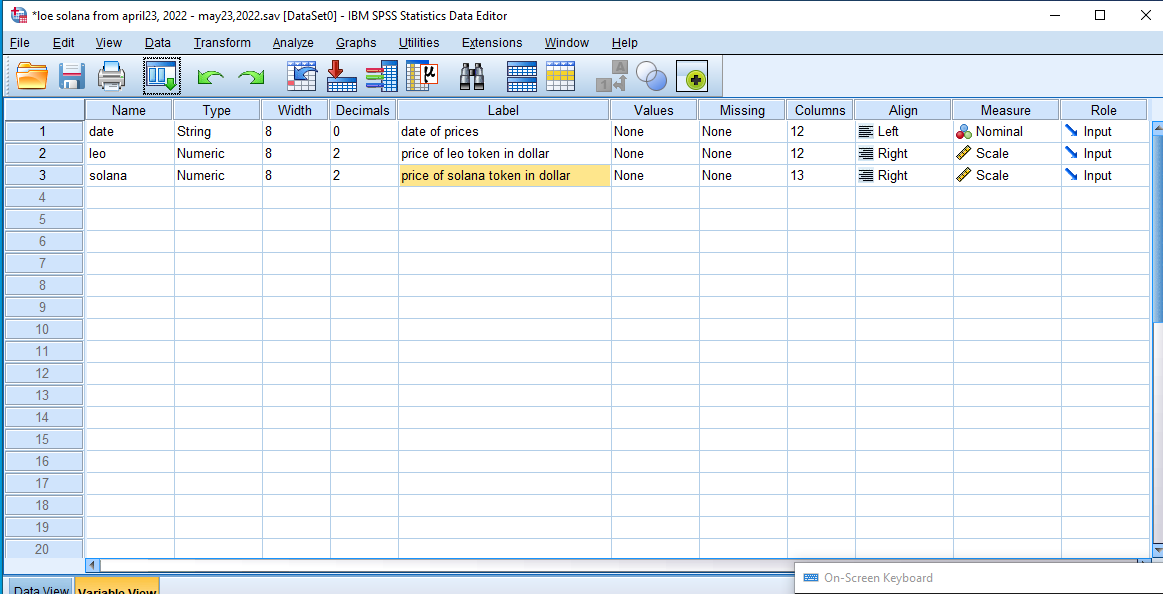Taken With Desktop Computer

This can be visualized below.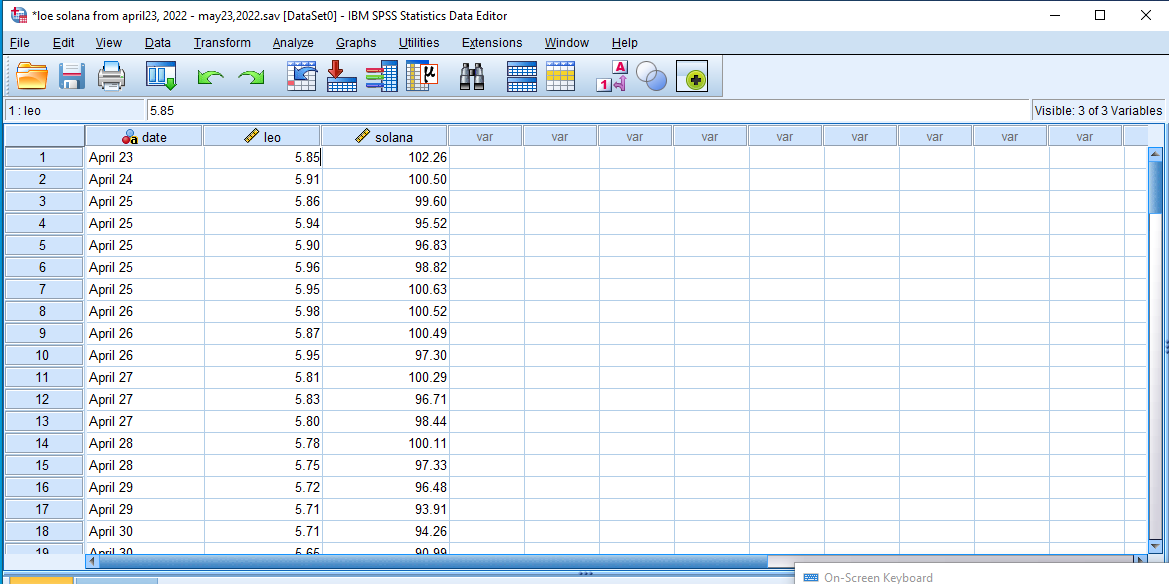Taken With Desktop Computer

As we seek to find the relationship between the price of Leo and Solana token, we shall visualize the direction of relationship using the scattered plot diagram.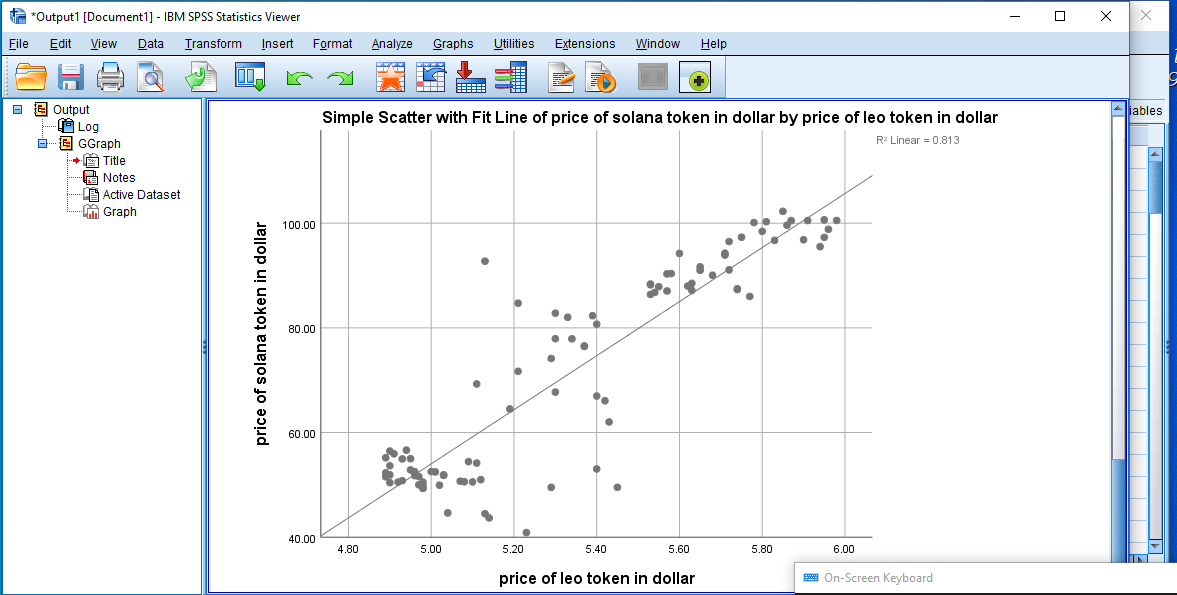Taken With Desktop Computer

It can be visualized that the direction of the relationship between the prices of both tokens is positive but we cant really tell how strong the positive relationship is.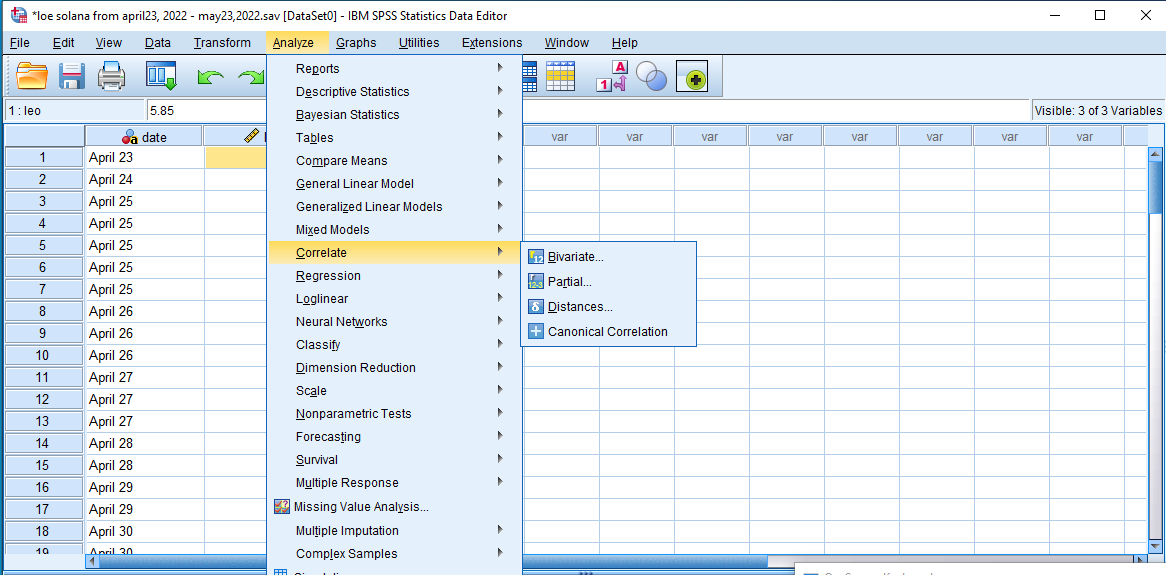Taken With Desktop Computer

We shall carryout a correlation test to proof the strength of positive relationship. The correlation test between both variables is as shown below.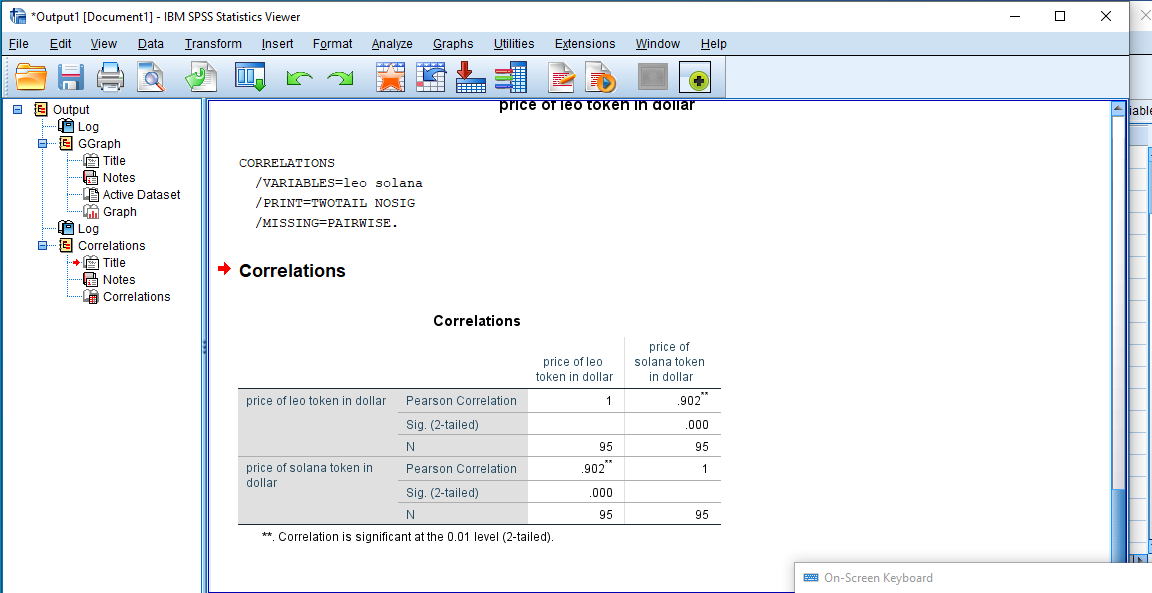Taken With Desktop Computer

It can be seen from the result of analysis between the price of Leo token and that of Solana that there is a maximum positive relationship considering 95 sample sizes.

This is to say as the price of Leo token goes high the price of Solana will also go high and vice versa.

Conclusion
Investors can invest in both tokens because there is a high positive relationship between both tokens (Leo and Solana) with a significance of 0.00 we can reject our hypothesis that there is no relationship between both tokens.

0
0
0.000Great post!

!1UP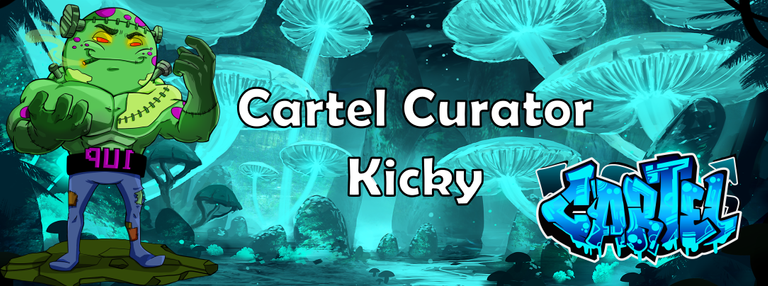0
0
0.000#### You have received a 1UP from @kwskicky!

The following @oneup-cartel family members will soon upvote your post:
`@leo-curator, @ctp-curator, @vyb-curator, @pob-curator, @neoxag-curator, @pal-curator`
And they will bring !PIZZA 🍕

Learn more about our delegation service to earn daily rewards. Join the family on Discord.

0
0
0.000